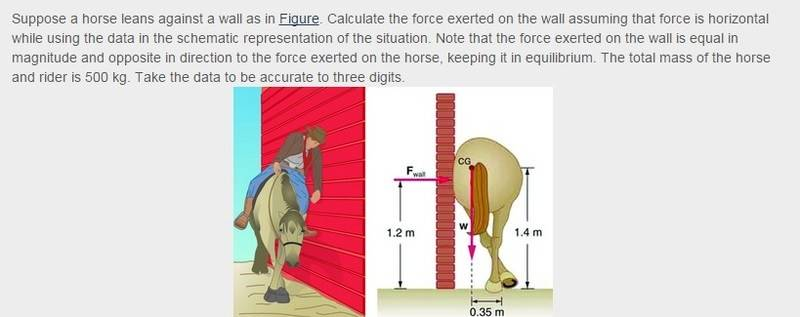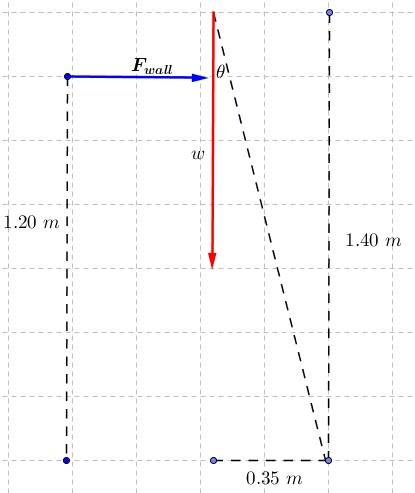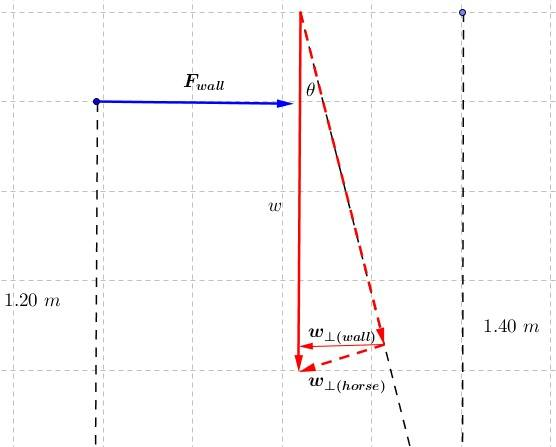# Stability: Leaning Horse against a Wall

## Homework StatementData:

##m = 500\ kg##
Distances given in the image.

## Homework Equations

##\tau = rF\sin(\theta)##

##F_{net} = ma##

## The Attempt at a Solution

It seems this problem is intended to be one where torque applies, but I don't see it in that way. And of course my answer doesn't agree with that given by the textbook :).

The diagram:According to my understanding, there is a component of the weight acting along the horse's body (the diagonal I've drawn) and another one perpendicular to its body, which is making it rotate to the left. I labeled the latter ##w_{\perp(horse)}##. The component of ##w_{\perp(horse)}## that lies perpendicular to the wall, I think, has the same magnitude that the force exerted by the wall on the horse. This is what I mean:Then:

##w_{\perp(horse)} = w\sin(\theta)##

##F_{wall} = w_{\perp(horse)}\cos(\theta)##

##F_{wall} = w\sin(\theta)\cos(\theta)##

And by Newton's Third Law, this is the force exerted on the wall.

The angle is found to be ##14.04º##, so

##F_{wall} = 500\ kg \cdot 9.80\ m/s^2 \cdot \sin(14.04º) \cdot \cos(14.04º) = 1.15 \times 10^3 N##

Maybe it should have a negative sign since it's in the opposite direction, but that is not what I'm worried about. The textbook's answer is ##1.43 \times 10^3 N##. I have no idea what I'm missing /:.

Thanks !!

Last edited:

PhanthomJay
Homework Helper
Gold Member
You are over complicating this problem and getting messed up. Sum torques about the horses feet. Torque of a force is force times perpendicular distance.

You are over complicating this problem and getting messed up. Sum torques about the horses feet. Torque of a force is force times perpendicular distance.

Ah, I see. I confused the perpendicular distance with the perpendicular component of the force (I've been doing so all the time :) ).

##\sum \tau = 0 = \tau_{ccw} - \tau_{cw}##

##\sum \tau = 0 = \tau_{weight} - \tau_{wall}##

##\tau_{wall} = \tau_{weight}##

##F_{wall} = \frac{500\ kg \cdot 9.80\ m/s^2 \cdot 0.35\ m}{1.20\ m}##

##F_{wall} = 1.43 \times 10^3\ N##

If I were to solve a similar problem where ##\sum F = 0##, I must then assume ##F_{wall} = friction ## and ##w = F_{normal}## about the horses feet, right? I want to be sure about it, because I also got confused with that and with the fact that the forces are acting along different heights/distances.

PhanthomJay
Homework Helper
Gold Member
If I were to solve a similar problem where ##\sum F = 0##, I must then assume ##F_{wall} = friction ## and ##w = F_{normal}## about the horses feet, right? I want to be sure about it, because I also got confused with that and with the fact that the forces are acting along different heights/distances.
Yes, that is correct. Normal force from ground acts up on the horses feet equal in magnitude to its weight, and the ground friction force on its feet acts left , equal in magnitude to the normal wall force.

Yes, that is correct. Normal force from ground acts up on the horses feet equal in magnitude to its weight, and the ground friction force on its feet acts left , equal in magnitude to the normal wall force.

Nice!

Thanks for helping me [: